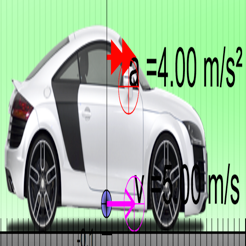## Screenshots

•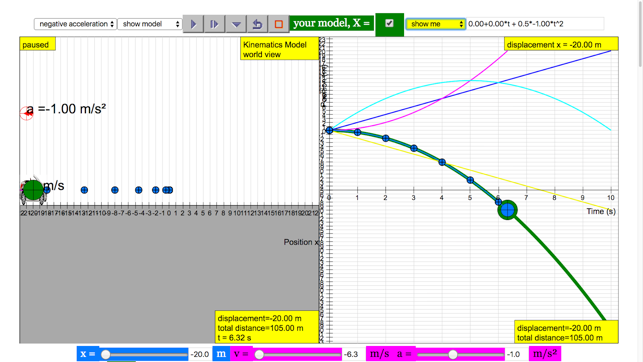•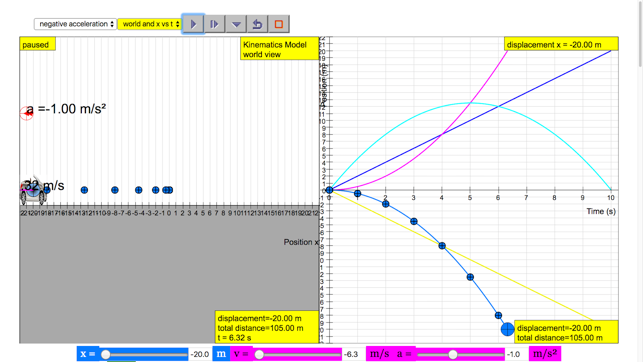•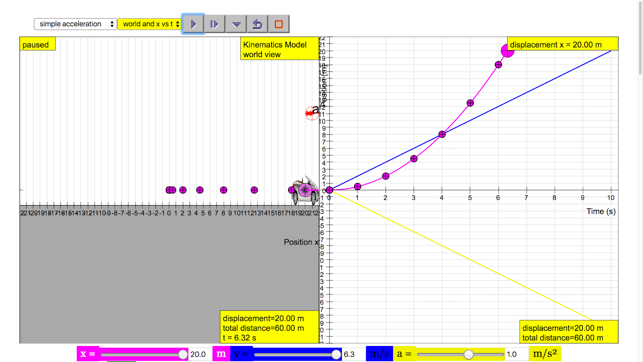•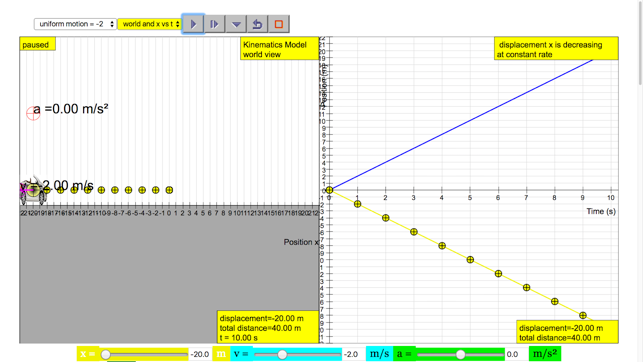•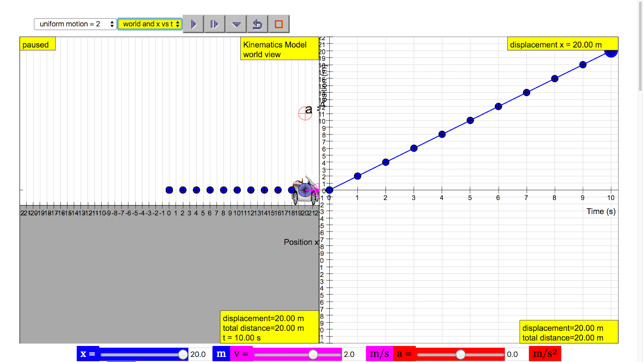## Description

an open source physics at Singapore simulation based on codes written by Félix Jesús Garcia Clemente, Francisco Esquembre and Loo Kang Wee.

more simulation are available on Support URL

Introduction
Topics

Kinematics
Speed, velocity and acceleration
Graphical analysis of motion

Description
This simulation has a drop-down menu for exploration of
(i) at rest use of progressive mathematical model is encouraged X = 0 for example
(ii) moving with uniform velocity, use of progressive mathematical model is encouraged for example X = 1*t for a constant velocity motion of v =1 m/s
(iii) moving with non-uniform velocity (eg, constant acceleration) use of progressive mathematical model is encouraged for example X = 0.5*1*t^2 for a constant acceleration motion of a =1 m/s^2
When only the velocity-time graph check-box is selected, it can be explored for the following cases too.
(i) at rest ,
(ii) moving with uniform velocity (eg, no acceleration)
(iii) moving with uniform acceleration (eg, constant acceleration = 9.81 m/s^2)
(iv) moving with non-uniform acceleration

Sample Learning Goals
(e) plot and interpret a displacement-time graph and a velocity-time graph
(f) deduce from the shape of a displacement-time graph when a body is:
(i) at rest example of progressive mathematical model is encouraged X = 0
(ii) moving with uniform velocity example of progressive mathematical model is encouraged X = 1*tf or a constant velocity motion of v =1 m/s
(iii) moving with non-uniform velocity example of X = 0.5*1*t^2 for a constant acceleration motion of a =1 m/s^2
(g) deduce from the shape of a velocity-time graph when a body is:
(i) at rest
(ii) moving with uniform velocity
(iii) moving with uniform acceleration
(iv) moving with non-uniform acceleration

Interesting Fact 1
Mystery could take the form of to describe it in an equation, called model.
Give the challenge to solve the mystery of a predictive equation that can be use to tell the future, more precisely the movement of a car, in a physics lesson.
Through the model selected by the students, it gives an indication of the students prior knowledge about what they know now, so that the teacher can understand the gaps of understanding for personalised mentoring

Interesting Fact 2
This app is produce real numbers to coincident with the real world data of gravity pull at 9.81 m/s^2 with some random errors simulating real data-logger results. There is a mathematical modelling pedagogy built into the simulation for students to make effort to represent the physics similar to Tracker's particle modeling.

Acknowledgement
My sincere gratitude for the tireless contributions of Francisco Esquembre, Fu-Kwun Hwang, Wolfgang Christian, Félix Jesús Garcia Clemente, Anne Cox, Andrew Duffy, Todd Timberlake and many more in the Open Source Physics community. I have designed much of the above based on their ideas and insights, and I thank the OSP community for which Singapore was honored with 2015-6 UNESCO King Hamad Bin Isa Al-Khalifa Prize for the Use of ICTs in Education.

## What’s New

Version 0.0.9

better zooming and drag features

## Information

Seller
loo kang wee
Size
13.6 MB
Category
Education
Compatibility

Requires iOS 8.0 or later. Compatible with iPhone, iPad, and iPod touch.

Languages

English

Age Rating
4+
Price
Free

## Supports

•